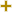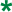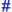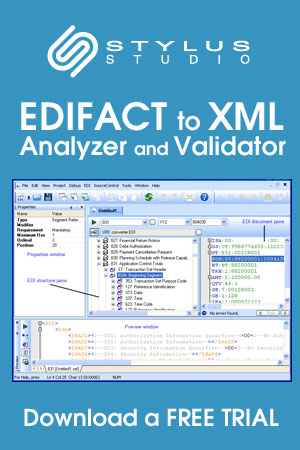Return to Stylus Studio EDIFACT D04A Code List page.

Formula sequence operand codeUN/CEFACT Revision 2004A Code ListRepr:an..17
Desc:Code specifying a specific type of operand within a formula sequence.
This table also occurs in the following versions of this standard:
D00A, D00B, D01A, D01B, D01C, D02A, D02B, D03A, D03B, D04A, D04B, D99A, D99B

 1 Plus The formula sequence operand is an addition. 2 Minus The formula sequence operand is a subtraction. 3 Multiply The formula sequence operand is a multiplication. 4 Divide The formula sequence operand is a division. 5 Exponent The formula sequence operand is an exponent.

Change indicators
plus signAn addition.
asteriskAddition/substraction/change to a code entry for a particular data element.
hash or pound signChanges to names.
vertical barChanges to text for descriptions, notes and functions.
minus signA deletion.
letter XMarked for deletion.

Usage indicatorsUsed in batch messages only.Common usage in both batch and interactive messages.Used in interactive messages only.

Return to Stylus Studio EDIFACT D04A Code List page.